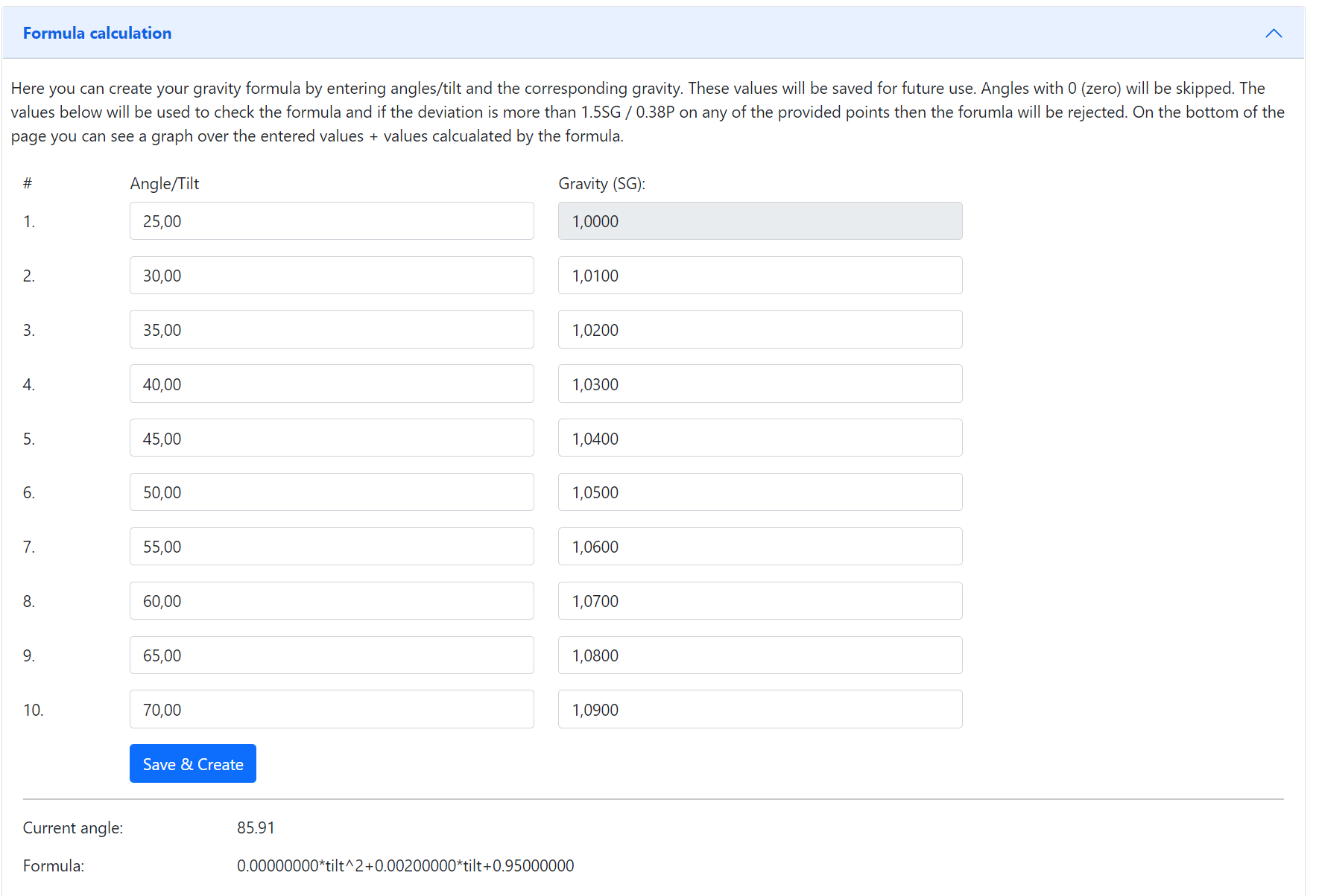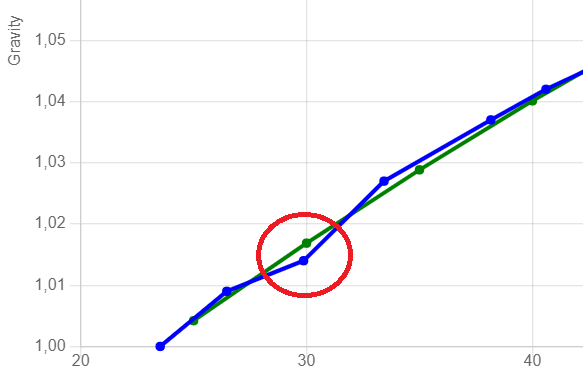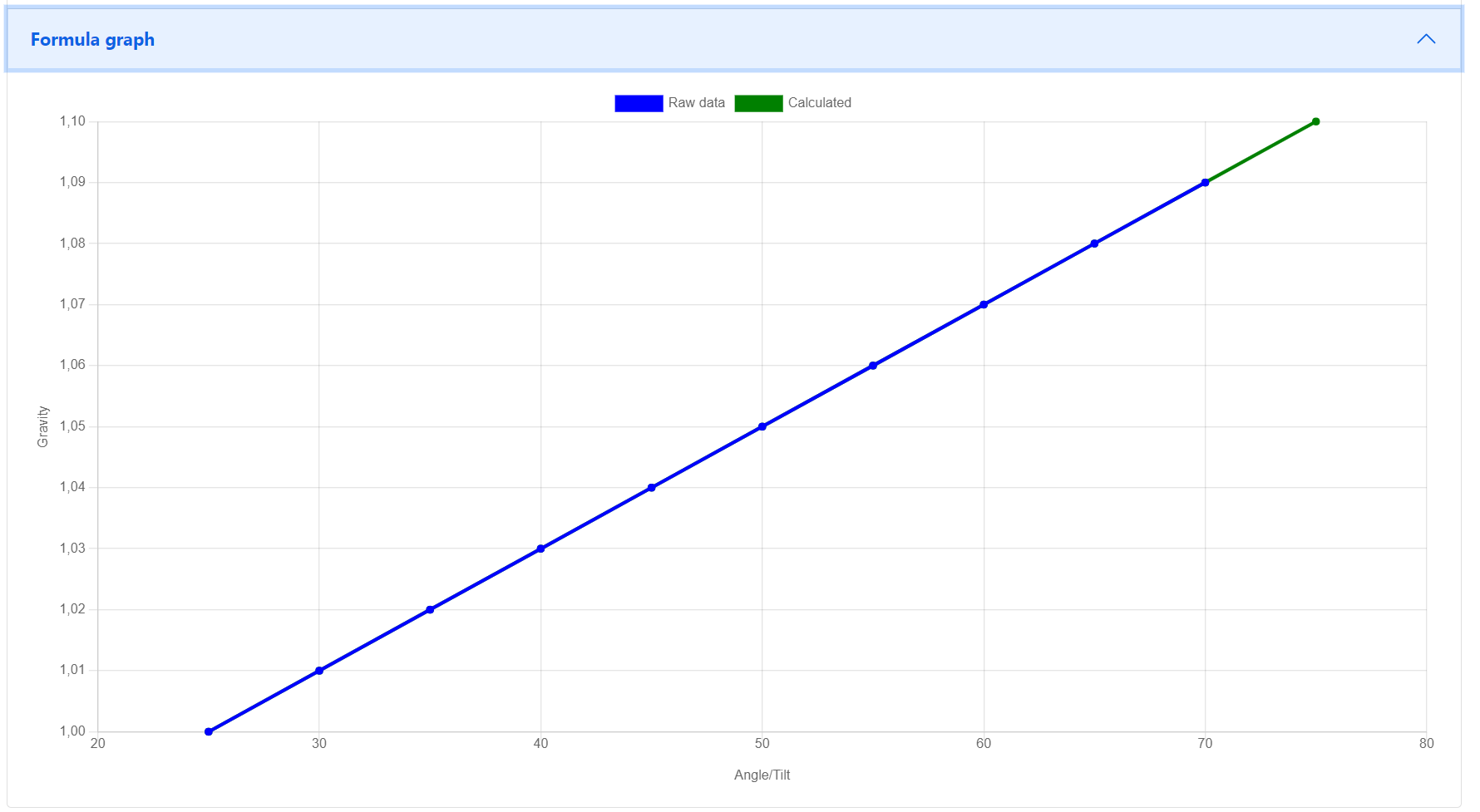# Create formula¶Here you can enter up to 10 values (angles + gravity) that is then used to create the formula. Angles equal to zero will be regarded as empty even if there is a gravity reading.

When you submit the values the device will try create a formula with increasing level of complexity. It will start with a order 2 formula and then try 3 and 4.

Once the formula has been created it will validate the formula against the supplied angles/gravity and if there is a too high difference, it will fail. You can adjust the sensitivity under advanced settings if you have issues.

Under the Error Log you will also find hints to what problem the formula creator encountered. Here is an example:

CALC: Validation failed on angle 33.430000, deviation too large 5.86, formula order 4

CALC: Validation failed on angle 33.430000, deviation too large 3.14, formula order 2

This means that the angle 33.43 had a deviation of 5.8 SG and since the default threshold is 3, it will fail. You can also see that it has failed on that point in both a order 2 and 4 formula.So in this case you can either increase the threshold or remove the angle that has an issue. You can also use the graph on the calibration page to identify angles that is probably not correct.Once the formula is created a graph over the entered values and a simulation of the formula will give you a nice overview on how the formula will work.